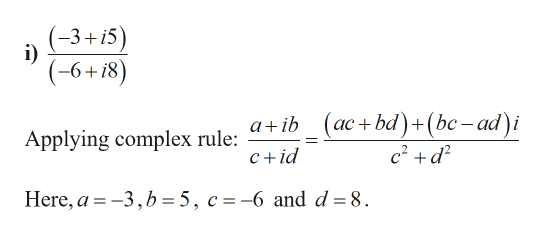# What would be the ratio of this equation (-3+i5)/(-6+i8)? & what would be the product of this equation (-4+i3)(-4-i3)

Question
5 views

What would be the ratio of this equation (-3+i5)/(-6+i8)? & what would be the product of this equation (-4+i3)(-4-i3)

check_circle

Step 1

Given:

Step 2

Calculation:help_outlineImage Transcriptionclose(-3+i5) (-6+18) (ас + bd) + (bc -ad)i c2 d2 a ib Applying complex rule: c+id Here, a-3,b = 5, c =-6 and d = 8 fullscreen
Step 3

### Want to see the full answer?

See Solution

#### Want to see this answer and more?

Solutions are written by subject experts who are available 24/7. Questions are typically answered within 1 hour.*

See Solution
*Response times may vary by subject and question.
Tagged in

### Complex Numbers